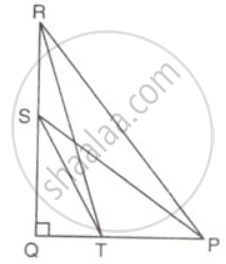# In the given figure, ∠Q = 90°, PS is a median om QR from P, and RT divides PQ in the ratio 1 : 2. Find: tan ∠ TSQ tan ∠ PRQ - Mathematics

Sum

In the given figure, ∠Q = 90°, PS is a median om QR from P, and RT divides PQ in the ratio 1 : 2. Find: ("tan" ∠"TSQ")/("tan"∠"PRQ")#### Solution

As PS is the median on QR from P.
∴ QS = SR
⇒ QR = 2QS
and RT divides PQ in the ratio 1 : 2
∴ QT = x and PT = 2x
⇒  PQ = 3x

("tan" ∠"TSQ")/("tan"∠"PRQ")

= ("QT"/"QS")/("PQ"/"QR")

= "QT"/"QS" xx "QR"/"PQ"

= x/"QS" xx (2"QS")/(3x)

= (2)/(3).

Concept: Reciprocal Relations
Is there an error in this question or solution?

#### APPEARS IN

Frank Class 9 Maths ICSE
Chapter 26 Trigonometrical Ratios
Exercise 26.1 | Q 19.2